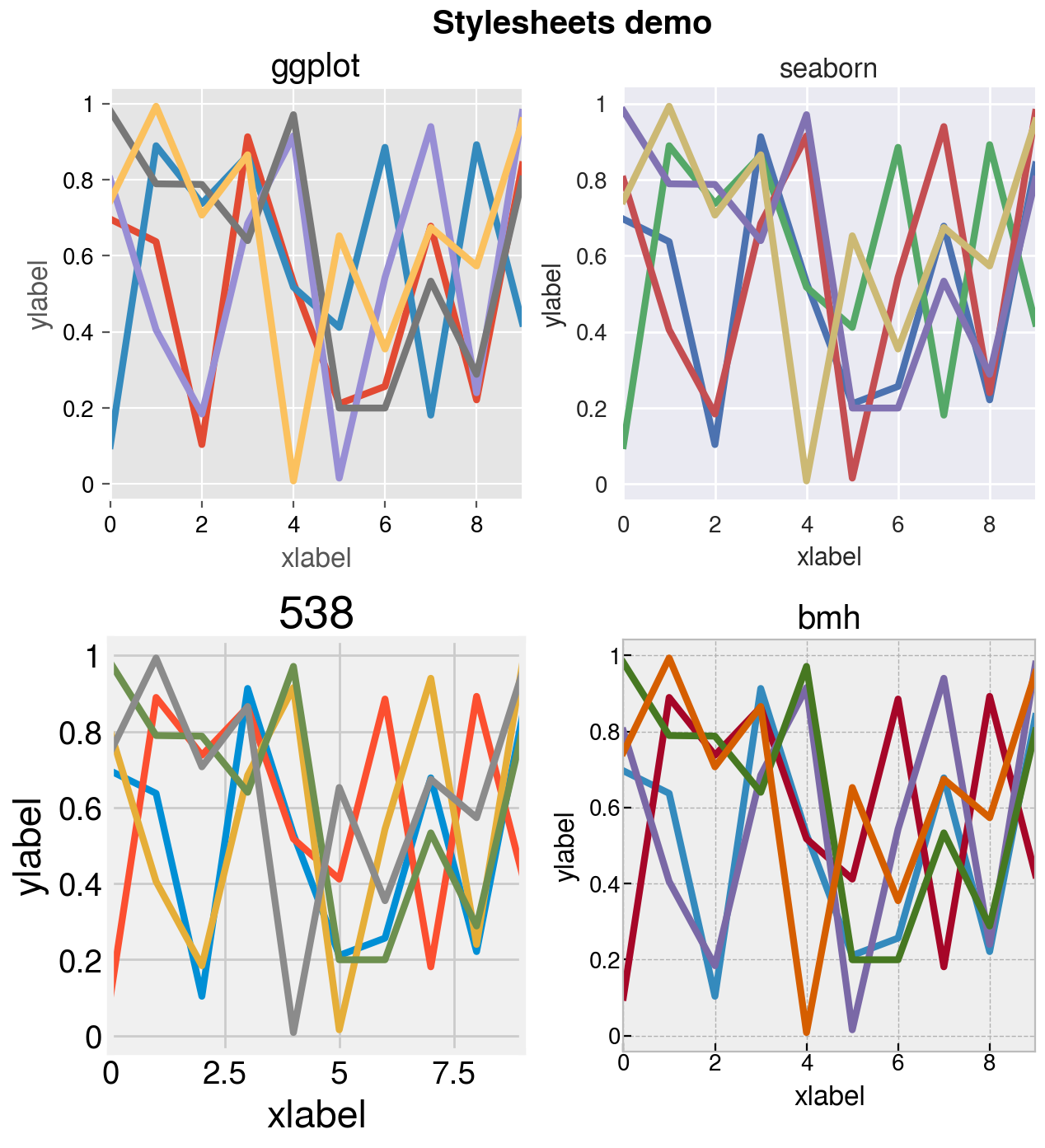# The basics¶

## Creating figures¶

ProPlot works by creating a proplot.figure.Figure subclass of the matplotlib figure class Figure, and a proplot.axes.Axes subclass of the matplotlib axes class Axes.

The subplots command is used to create ProPlot figures. Modeled after matplotlib.pyplot.subplots, it generates a proplot.figure.Figure instance filled with proplot.axes.Axes instances. subplots can be used as follows:

Figures can be saved with proplot.figure.Figure.save (or, equivalently, savefig). Tildes in the filename are expanded with os.path.expanduser. In the below examples, we create a few simple figures with subplots. See the next sections for details.

Note

ProPlot changes the default rc[‘figure.facecolor’] so that the figure backgrounds shown by the matplotlib backend are gray (the rc[‘savefig.facecolor’] applied to saved figures is still white). This can be helpful when designing figures. ProPlot also controls the appearence of figures in Jupyter notebooks using the new rc.inlinefmt setting, which is passed to config_inline_backend on import. This imposes a higher-quality default “inline” format and disables the backend-specific settings InlineBackend.rc and InlineBackend.print_figure_kwargs, ensuring that the figures you save look identical to the figures displayed by the backend.

ProPlot also changes the default rc[‘savefig.format’] from PNG to PDF for the following reasons:

1. Vector graphic formats are infinitely scalable.

2. Vector graphic formats are preferred by academic journals.

3. Nearly all academic journals accept figures in the PDF format alongside the EPS format.

4. The EPS format is outdated and does not support transparent graphic elements.

In case you do need a raster format like PNG, ProPlot increases the default rc[‘savefig.dpi’] to 1000 dots per inch, which is recommended by most journals as the minimum resolution for rasterized figures containing lines and text. See the configuration section for how to change these settings.

Warning

ProPlot enables “axis sharing” by default. This lets subplots in the same row or column share the same axis limits, scales, ticks, and labels. This is often convenient, but may be annoying for some users. To keep this feature turned off, simply change the default settings with e.g. pplt.rc.update(share=False, span=False). See the axis-sharing section for details.

:

# Sample data
import numpy as np
state = np.random.RandomState(51423)
data = 2 * (state.rand(100, 5) - 0.5).cumsum(axis=0)

:

# Single subplot
import proplot as pplt
fig, ax = pplt.subplots()
ax.plot(data, lw=2)
ax.format(suptitle='Single subplot', xlabel='x axis', ylabel='y axis')
# Save the figure
fig.save('~/test1.png')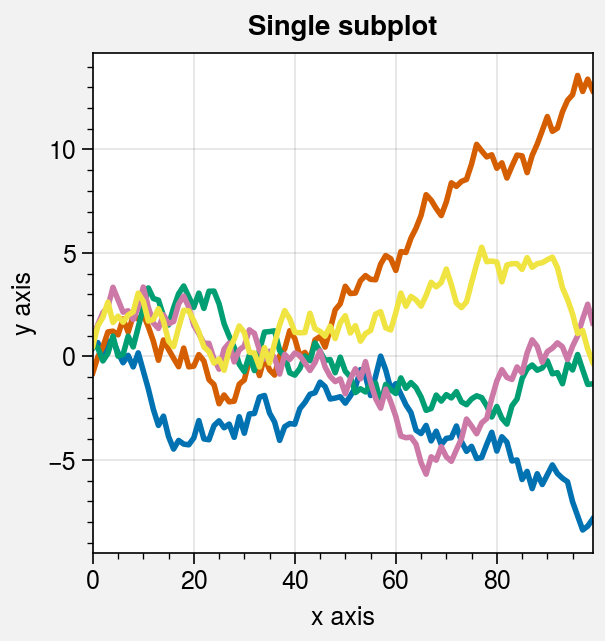:

# Simple subplot grid
import proplot as pplt
fig, axs = pplt.subplots(ncols=2)
axs.plot(data, lw=2)
axs.format(xticks=20, xtickminor=False)
axs.format(
suptitle='Simple subplot grid', title='Title',
xlabel='x axis', ylabel='y axis'
)
# Save the figure
fig.save('~/test2.png')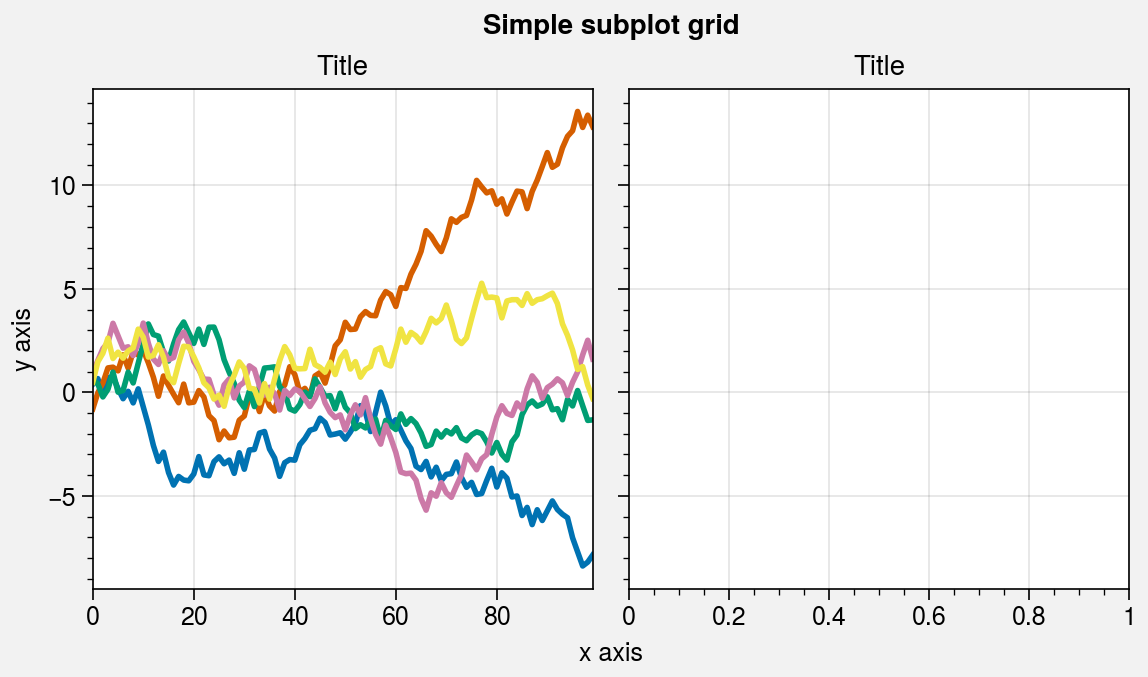:

# Complex grid
import proplot as pplt
array = [  # the "picture" (0 == nothing, 1 == subplot A, 2 == subplot B, etc.)
[1, 1, 2, 2],
[0, 3, 3, 0],
]
fig, axs = pplt.subplots(array, refwidth=1.8)
axs.format(
abc=True, abcloc='ul', suptitle='Complex subplot grid',
xlabel='xlabel', ylabel='ylabel'
)
axs.plot(data, lw=2)
# Save the figure
fig.save('~/test3.png')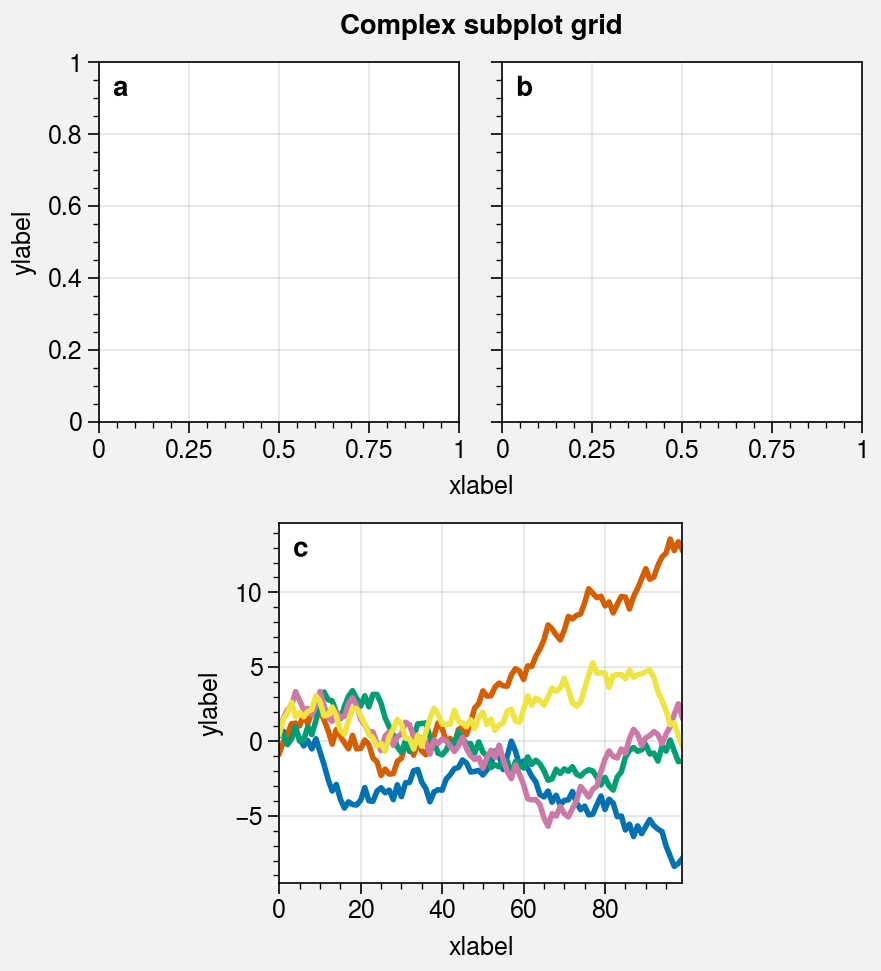:

# Really complex grid
import proplot as pplt
array = [  # the "picture" (1 == subplot A, 2 == subplot B, etc.)
[1, 1, 2],
[1, 1, 6],
[3, 4, 4],
[3, 5, 5],
]
fig, axs = pplt.subplots(array, figwidth=5, span=False)
axs.format(
suptitle='Really complex subplot grid',
xlabel='xlabel', ylabel='ylabel', abc=True
)
axs.plot(data, lw=2)
# Save the figure
fig.save('~/test4.png')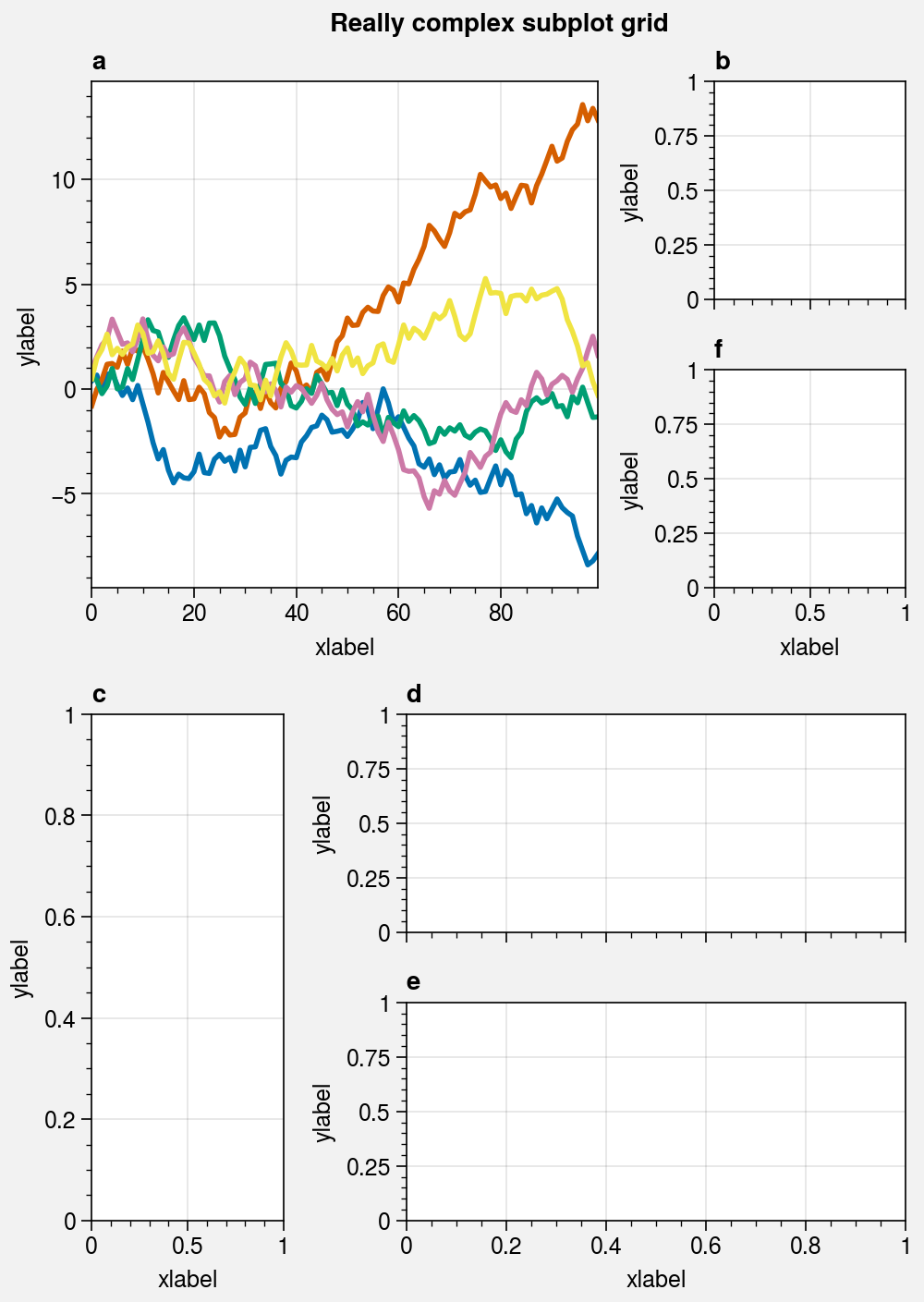## Plotting stuff¶

Matplotlib has two different interfaces: an object-oriented interface and a MATLAB-style pyplot interface (which uses the object-oriented interface internally). Plotting with ProPlot is just like plotting with matplotlib’s object-oriented interface. Proplot builds upon the matplotlib constructs of the Figure and the Axes by adding new commands and adding new features to existing commands. These additions do not change the usage or syntax of existing commands, which means a shallow learning curve for the average matplotlib user.

In the below example, we create a 4-panel figure with the familiar matplotlib commands plot, scatter, pcolormesh, and contourf. See the 1d plotting and 2d plotting sections for details on the features added by ProPlot.

:

import proplot as pplt
import numpy as np

# Sample data
N = 20
state = np.random.RandomState(51423)
data = N + (state.rand(N, N) - 0.55).cumsum(axis=0).cumsum(axis=1)

# Example plots
cycle = pplt.Cycle('greys', left=0.2, N=5)
fig, axs = pplt.subplots(ncols=2, nrows=2, figwidth=5, share=0)
axs.plot(data[:, :5], linewidth=2, linestyle='--', cycle=cycle)
axs.scatter(data[:, :5], marker='x', cycle=cycle)
axs.pcolormesh(data, cmap='greys')
m = axs.contourf(data, cmap='greys')
axs.format(
abc=True, abcstyle='a.', titleloc='l', title='Title',
xlabel='xlabel', ylabel='ylabel', suptitle='Quick plotting demo'
)
fig.colorbar(m, loc='b', label='label')

:

<matplotlib.colorbar.Colorbar at 0x7f56edce3af0>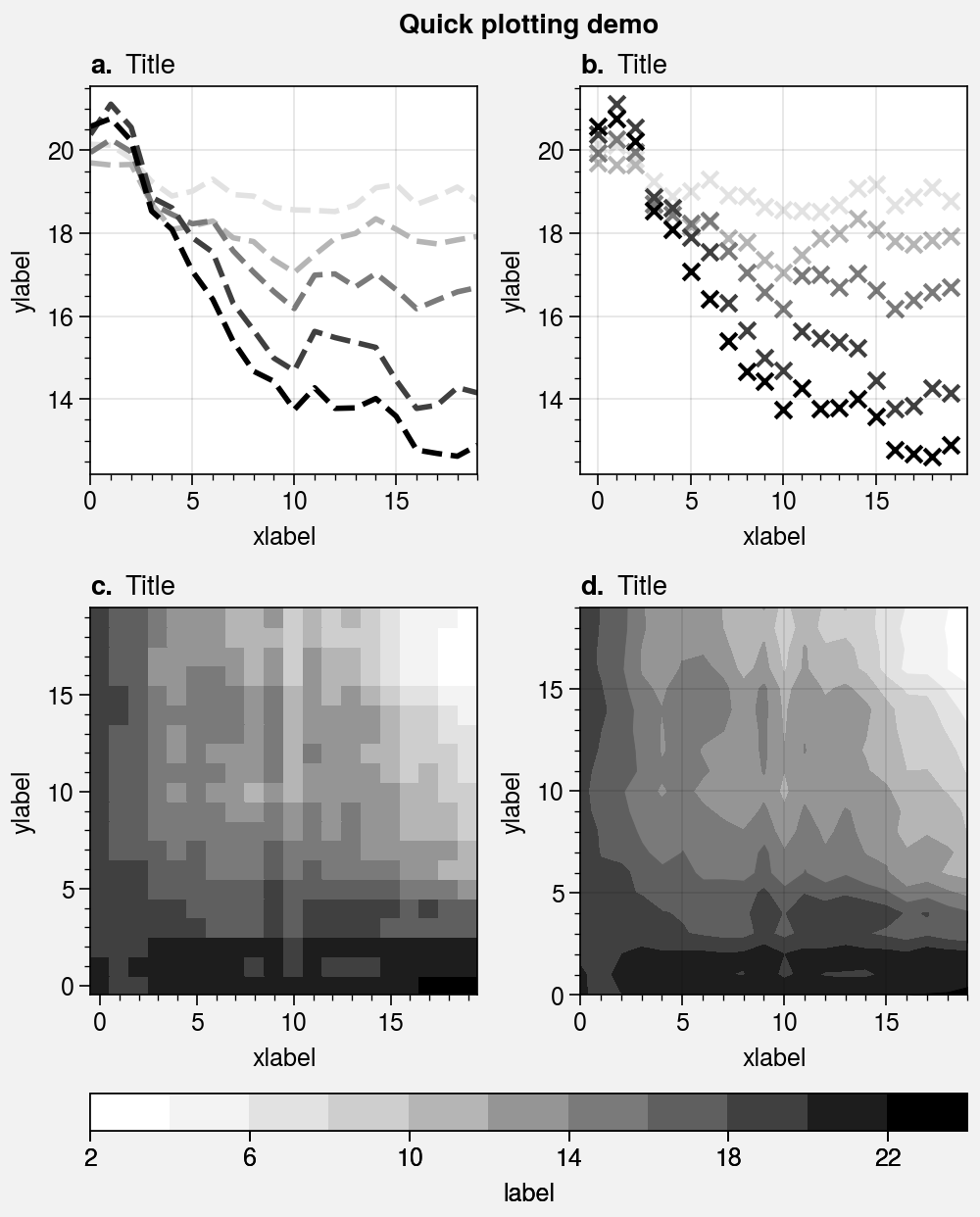## Formatting stuff¶

Every Axes returned by subplots has a format method. This is your one-stop-shop for changing axes settings. Keyword arguments passed to format are interpreted as follows:

1. Any keyword matching the name of an rc setting is used to update the axes. If the name has “dots”, you can omit them (e.g., titleloc='left' changes the rc[‘title.loc’] property). See the configuration section for details.

2. Valid keywords arguments are passed to proplot.axes.CartesianAxes.format, proplot.axes.PolarAxes.format, or proplot.axes.GeoAxes.format. These change settings that are specific to the axes type. For example:

1. Remaining keyword arguments are passed to the base proplot.axes.Axes.format method. Axes is the base class for all other axes classes. This changes things that are the same for all axes types, like titles and a-b-c subplot labels (e.g., title='Title').

The format methods let you use simple shorthands for changing all kinds of settings at once, instead of one-liner setter methods like ax.set_title() and ax.set_xlabel(). They are also integrated with the Locator, Formatter, and Scale constructor functions (see this section).

The below example shows the many different keyword arguments accepted by format, and demonstrates how format can be used to succinctly and efficiently customize your plots.

:

import proplot as pplt
import numpy as np
fig, axs = pplt.subplots(ncols=2, nrows=2, share=0, tight=True, refwidth=2)
state = np.random.RandomState(51423)
N = 60
x = np.linspace(1, 10, N)
y = (state.rand(N, 5) - 0.5).cumsum(axis=0)
axs.plot(x, y, linewidth=1.5)
axs.format(
suptitle='Format command demo',
abc=True, abcloc='ul', abcstyle='A.',
title='Main', ltitle='Left', rtitle='Right',  # different titles
ultitle='Title 1', urtitle='Title 2', lltitle='Title 3', lrtitle='Title 4',
toplabels=('Column 1', 'Column 2'),
leftlabels=('Row 1', 'Row 2'),
xlabel='x-axis', ylabel='y-axis',
xscale='log',
xlim=(1, 10), xticks=1,
ylim=(-3, 3), yticks=pplt.arange(-3, 3),
yticklabels=('a', 'bb', 'c', 'dd', 'e', 'ff', 'g'),
ytickloc='both', yticklabelloc='both',
xtickdir='inout', xtickminor=False, ygridminor=True,
)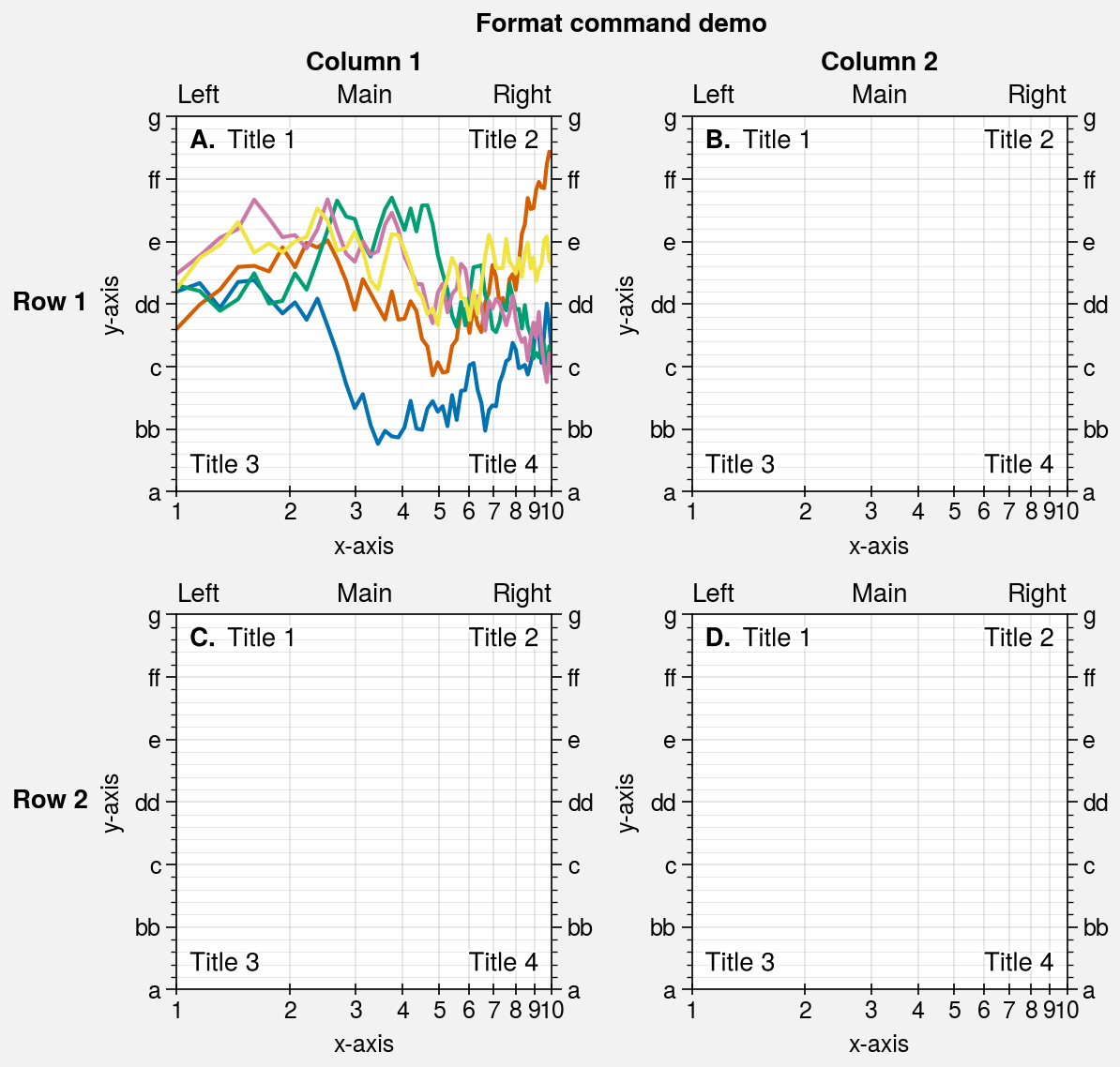## Subplot containers¶

matplotlib.pyplot.subplots returns a 2D ndarray for figures with more than one column and row, a 1D ndarray for single-row or column figures, or a lone Axes instance for single-subplot figures. By contrast, proplot.ui.subplots returns a SubplotsContainer that unifies these three possible return values:

• SubplotsContainer permits 2D indexing, e.g. axs[1, 0]. Since subplots can generate figures with arbitrarily complex subplot geometry, this 2D indexing is useful only when the arrangement happens to be a clean 2D matrix.

• SubplotsContainer permits 1D indexing, e.g. axs. The default order can be switched from row-major to column-major by passing order='F' to subplots.

• When it is singleton, SubplotsContainer behaves like a scalar. So when you make a single axes with fig, axs = pplt.subplots(), axs.method(...) is equivalent to axs.method(...).

SubplotsContainer is especially useful because it lets you call Axes methods simultaneously for all subplots in the container. In the below example, we use the SubplotsContainer returned by subplots with the proplot.axes.Axes.format command to format several subplots at once.

:

import proplot as pplt
import numpy as np
state = np.random.RandomState(51423)
fig, axs = pplt.subplots(ncols=4, nrows=4, refwidth=1.2)
axs.format(
xlabel='xlabel', ylabel='ylabel', suptitle='SubplotsContainer demo',
grid=False, xlim=(0, 50), ylim=(-4, 4)
)

# Various ways to select subplots in the container
axs[:, 0].format(facecolor='blush', color='gray7', linewidth=1)
axs[0, :].format(facecolor='sky blue', color='gray7', linewidth=1)
axs.format(color='black', facecolor='gray5', linewidth=1.4)
axs[1:, 1:].format(facecolor='gray1')
for ax in axs[1:, 1:]:
ax.plot((state.rand(50, 5) - 0.5).cumsum(axis=0), cycle='Grays', lw=2)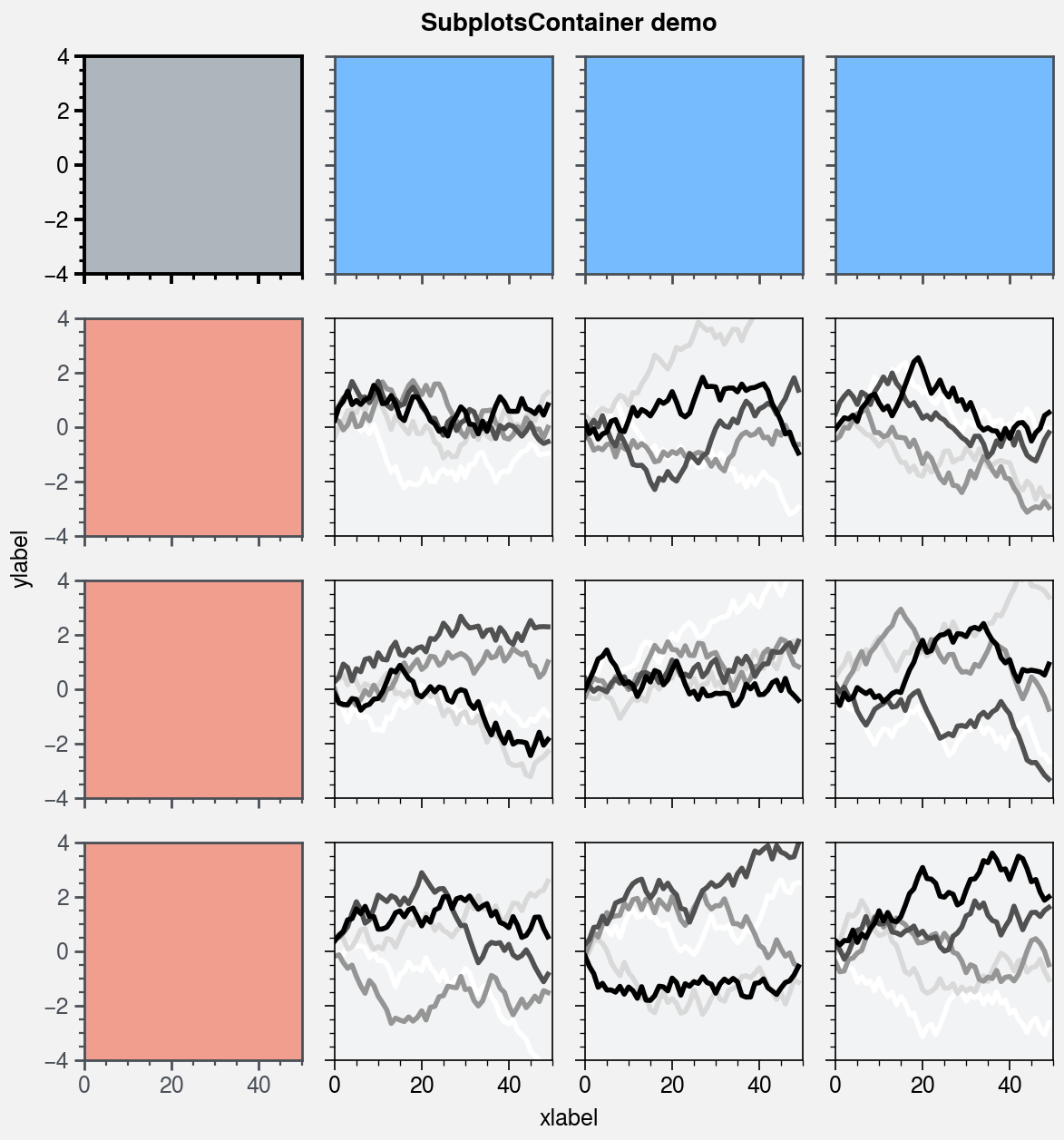## Settings and styles¶

A special object named rc is created whenever you import ProPlot. rc is similar to the matplotlib rcParams dictionary, but can be used to change both matplotlib settings and ProPlot settings. rc also provides a style parameter that can be used to switch between matplotlib stylesheets. See the configuration section for details.

To modify a setting for just one subplot, you can pass it to the proplot.axes.Axes.format method. To temporarily modify setting(s) for a block of code, use context. To modify setting(s) for the entire python session, just assign it to the rc object or use update. To reset everything to the default state, use reset. See the below example.

:

import proplot as pplt
import numpy as np

# Update global settings in several different ways
pplt.rc.cycle = 'colorblind'
pplt.rc.color = 'gray6'
pplt.rc.update({'fontname': 'Source Sans Pro', 'fontsize': 11})
pplt.rc['figure.facecolor'] = 'gray3'
pplt.rc.axesfacecolor = 'gray4'
# pplt.rc.save()  # save the current settings to ~/.proplotrc

# Apply settings to figure with context()
with pplt.rc.context({'suptitle.size': 13}, toplabelcolor='gray6', linewidth=1.5):
fig, axs = pplt.subplots(ncols=2, figwidth=6, sharey=2, span=False)

# Plot lines
N, M = 100, 6
state = np.random.RandomState(51423)
values = np.arange(1, M + 1)
for i, ax in enumerate(axs):
data = np.cumsum(state.rand(N, M) - 0.5, axis=0)
lines = ax.plot(data, linewidth=3, cycle='Grays')

# Apply settings to axes with format()
axs.format(
grid=False, xlabel='x label', ylabel='y label',
toplabels=('Column 1', 'Column 2'),
suptitle='Rc settings demo',
suptitlecolor='gray7',
abc=True, abcloc='l', abcstyle='(A)',
title='Title', titleloc='r', titlecolor='gray7'
)
ay = axs[-1].twinx()
ay.format(ycolor='red', linewidth=1.5, ylabel='secondary axis')
ay.plot((state.rand(100) - 0.2).cumsum(), color='r', lw=3)

# Reset persistent modifications from head of cell
pplt.rc.reset()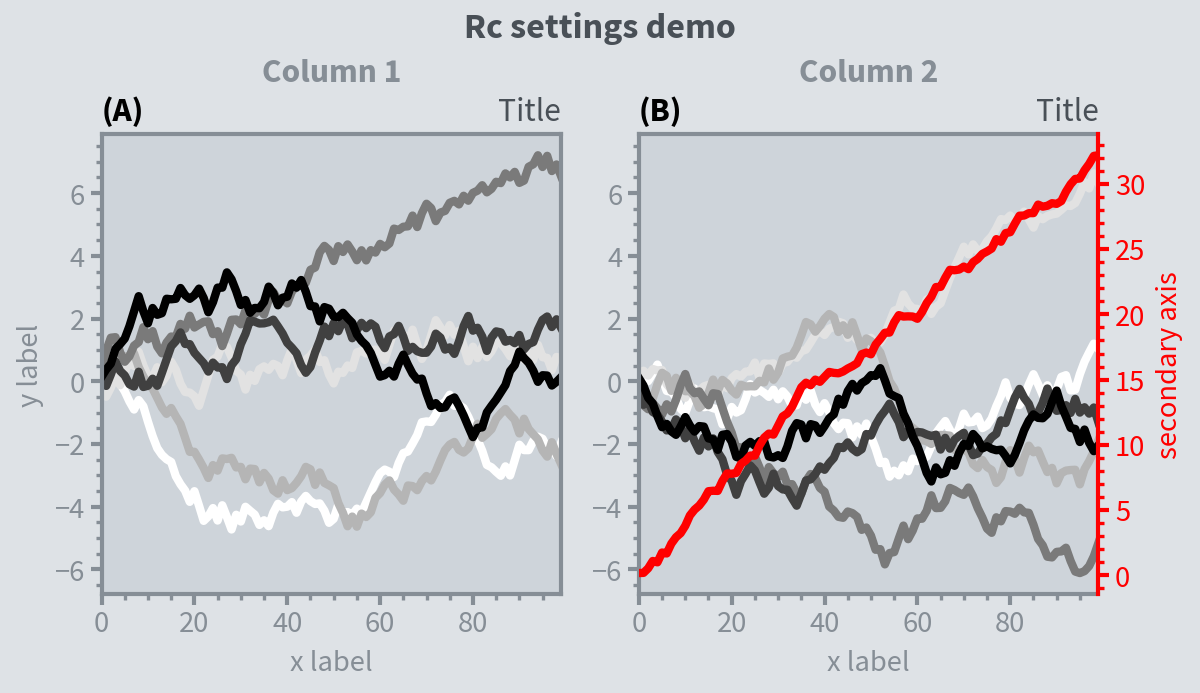:

import proplot as pplt
import numpy as np
# pplt.rc.style = 'style'  # set the style everywhere

# Sample data
state = np.random.RandomState(51423)
data = state.rand(10, 5)

# Set up figure
fig, axs = pplt.subplots(ncols=2, nrows=2, span=False, share=False)
axs.format(suptitle='Stylesheets demo')
styles = ('ggplot', 'seaborn', '538', 'bmh')

# Apply different styles to different axes with format()
for ax, style in zip(axs, styles):
ax.format(style=style, xlabel='xlabel', ylabel='ylabel', title=style)
ax.plot(data, linewidth=3)